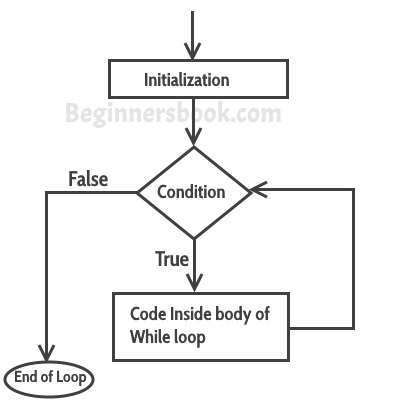C – while loop in C programming

A loop is used for executing a block of statements repeatedly until a given condition returns false. In the previous tutorial we learned for loop. In this post we will learn while loop in C.

while loop

Syntax of while loop:

while (condition test)
{
//Statements to be executed repeatedly
// Increment (++) or Decrement (--) Operation
}

Flow Diagram of while loopUse of Logical operators in while loop

Just like relational operators (<, >, >=, <=, ! =, ==), we can also use logical operators in while loop. The following scenarios are valid :

while(num1<=10 && num2<=10)

-using AND(&&) operator, which means both the conditions should be true.

while(num1<=10||num2<=10)

– OR(||) operator, this loop will run until both conditions return false.

while(num1!=num2 &&num1 <=num2)

– Here we are using two logical operators NOT (!) and AND(&&).

while(num1!=10 ||num2>=num1)